Published

# MEGR 3171 Lake Data Project

Team 19 (Daniel Shirilla, Dylan McClintock, River Issacsson-Savela)

BeginnerWork in progress4 hours61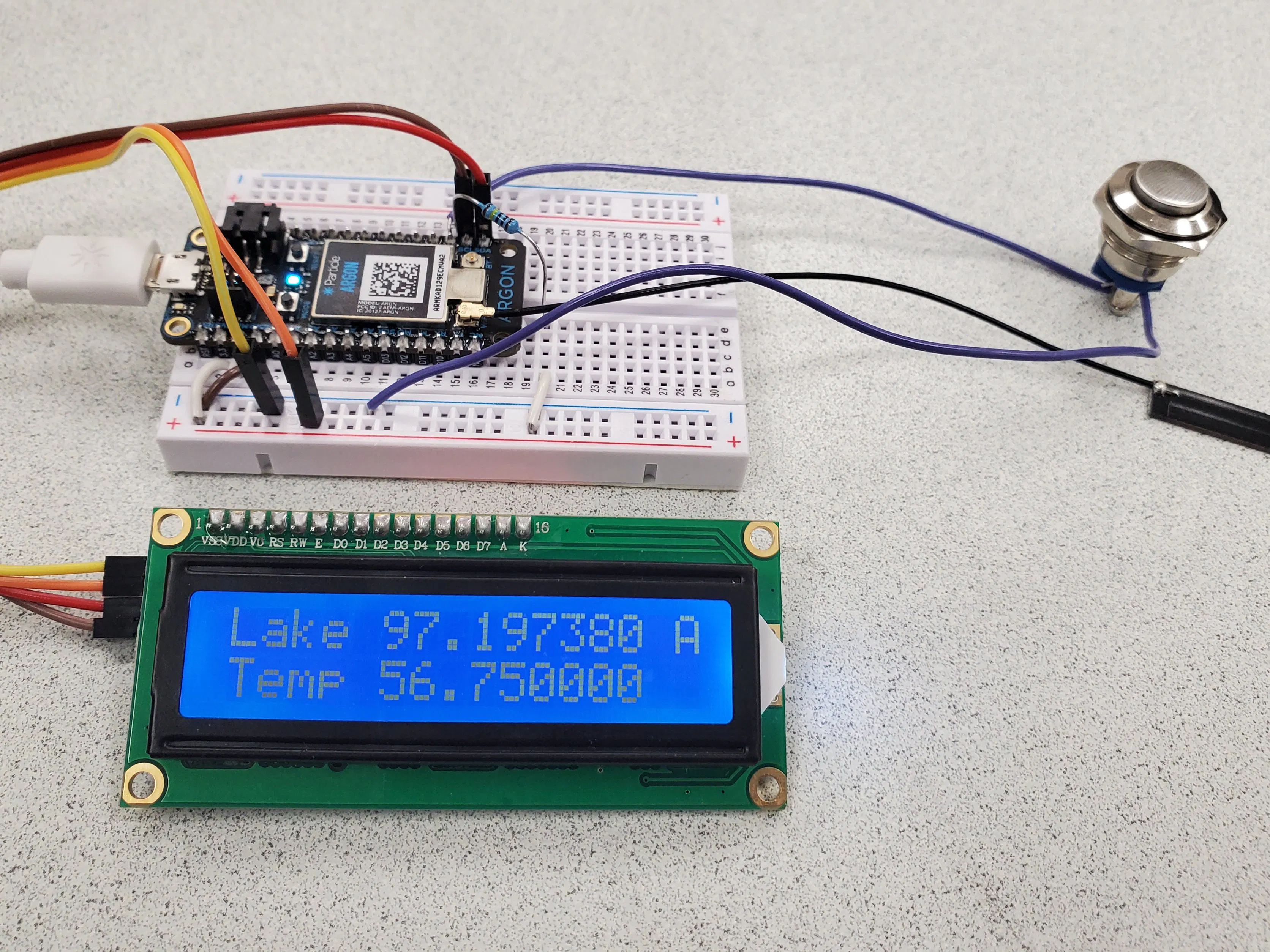## Things used in this project

### Hardware components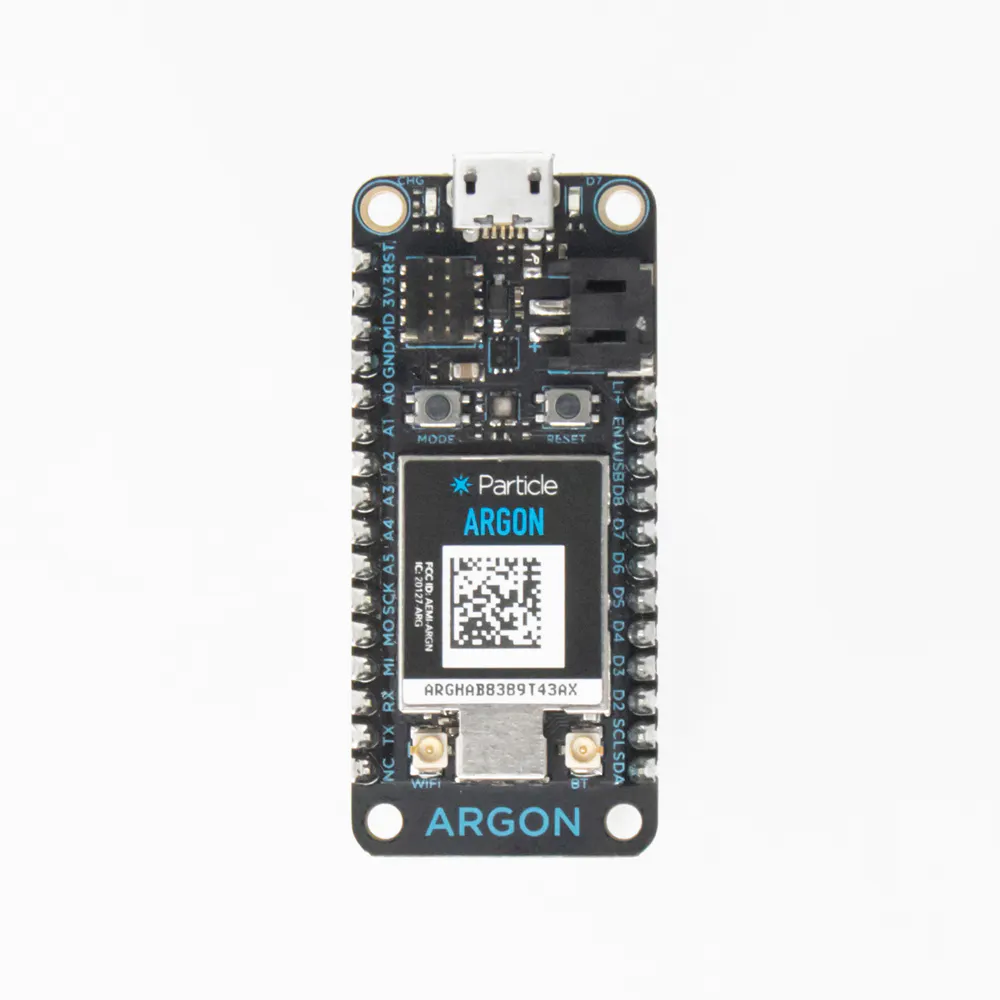Particle Argon
×3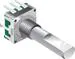Rotary Encoder with Push-Button
×1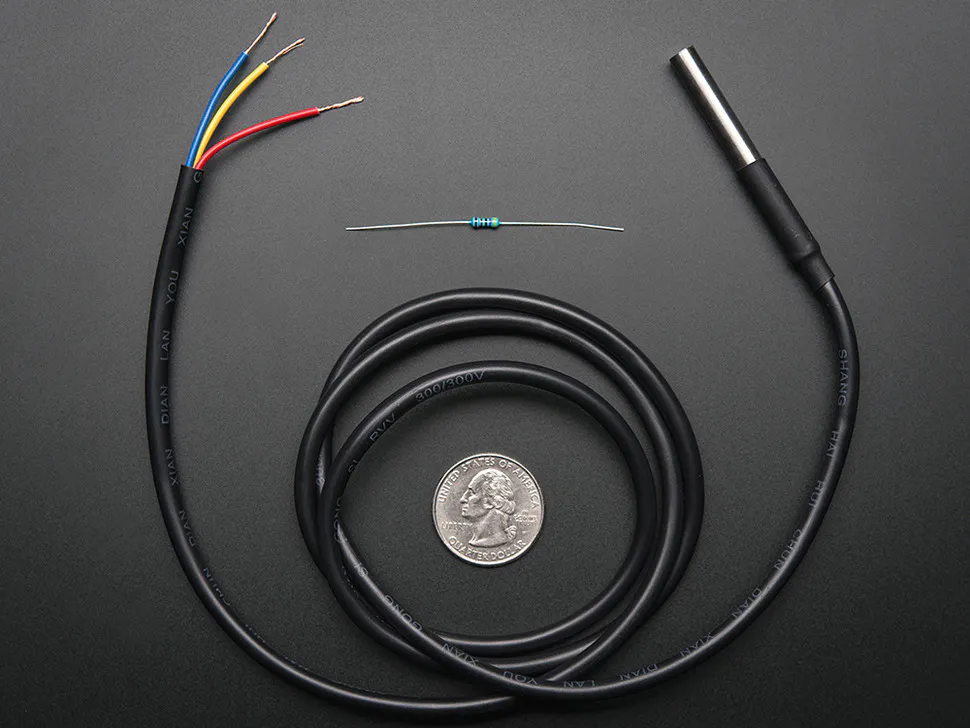Adafruit Waterproof DS18B20 Digital temperature sensor
×1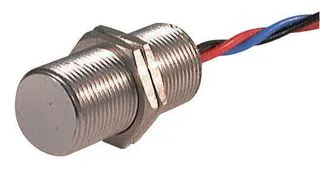Hall Effect Position Sensor, 4.5 to 24 Vdc
×1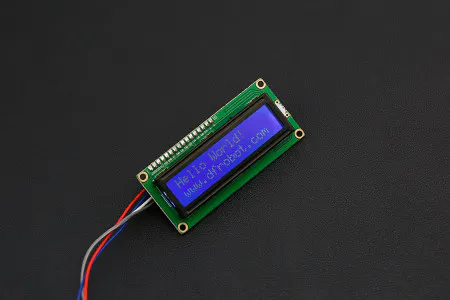DFRobot I2C 16x2 Arduino LCD Display Module
×1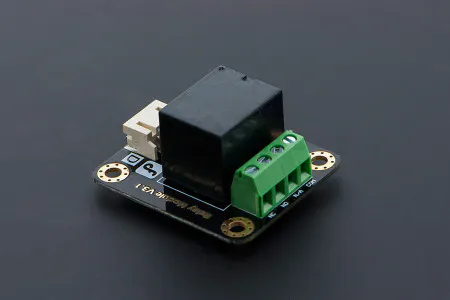DFRobot Gravity: Digital 5A Relay Module
×1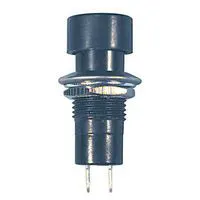Pushbutton Switch, Momentary
×1

## Schematics

### Sensor Argon Schematic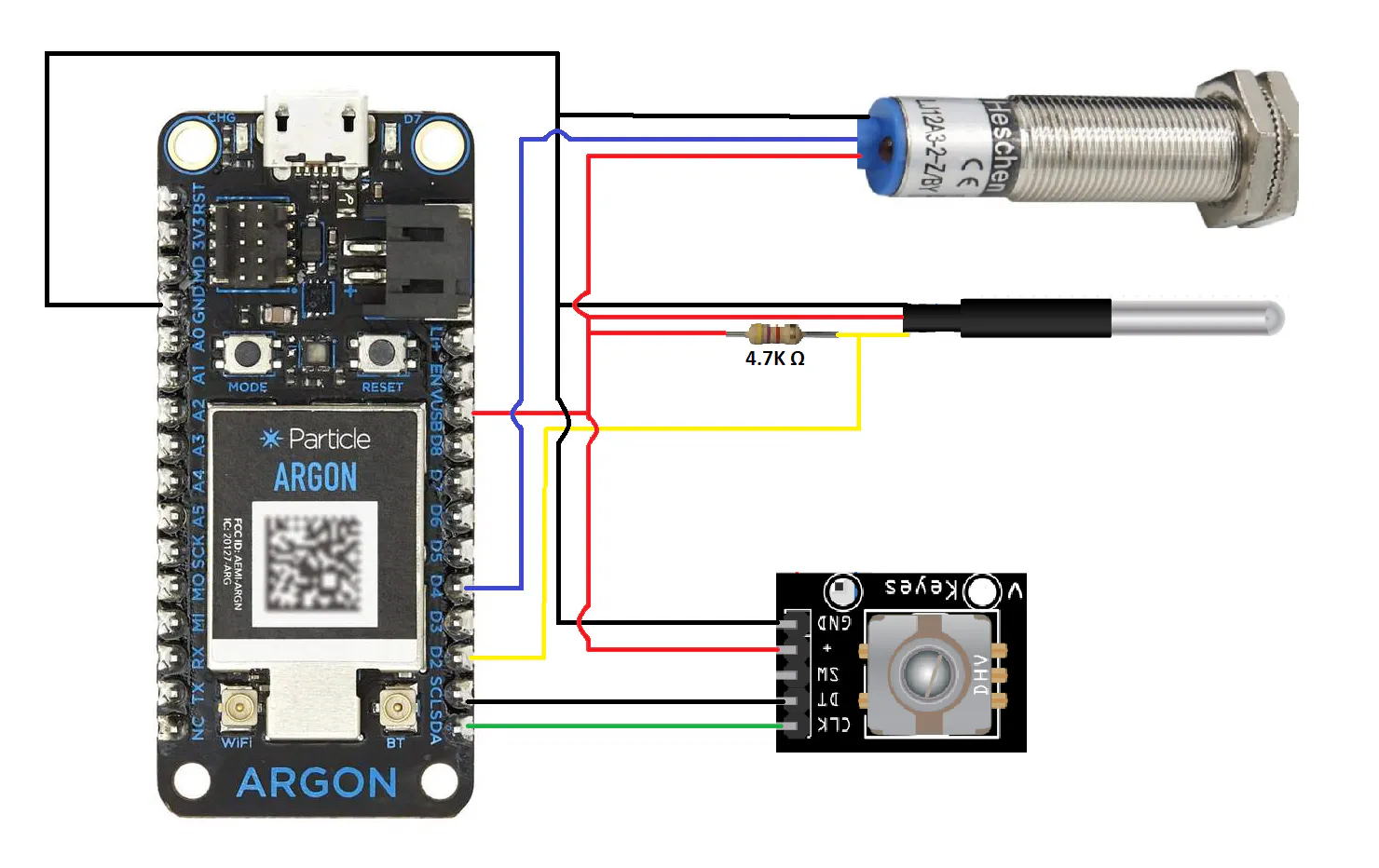### Irrigation System Argon Schematic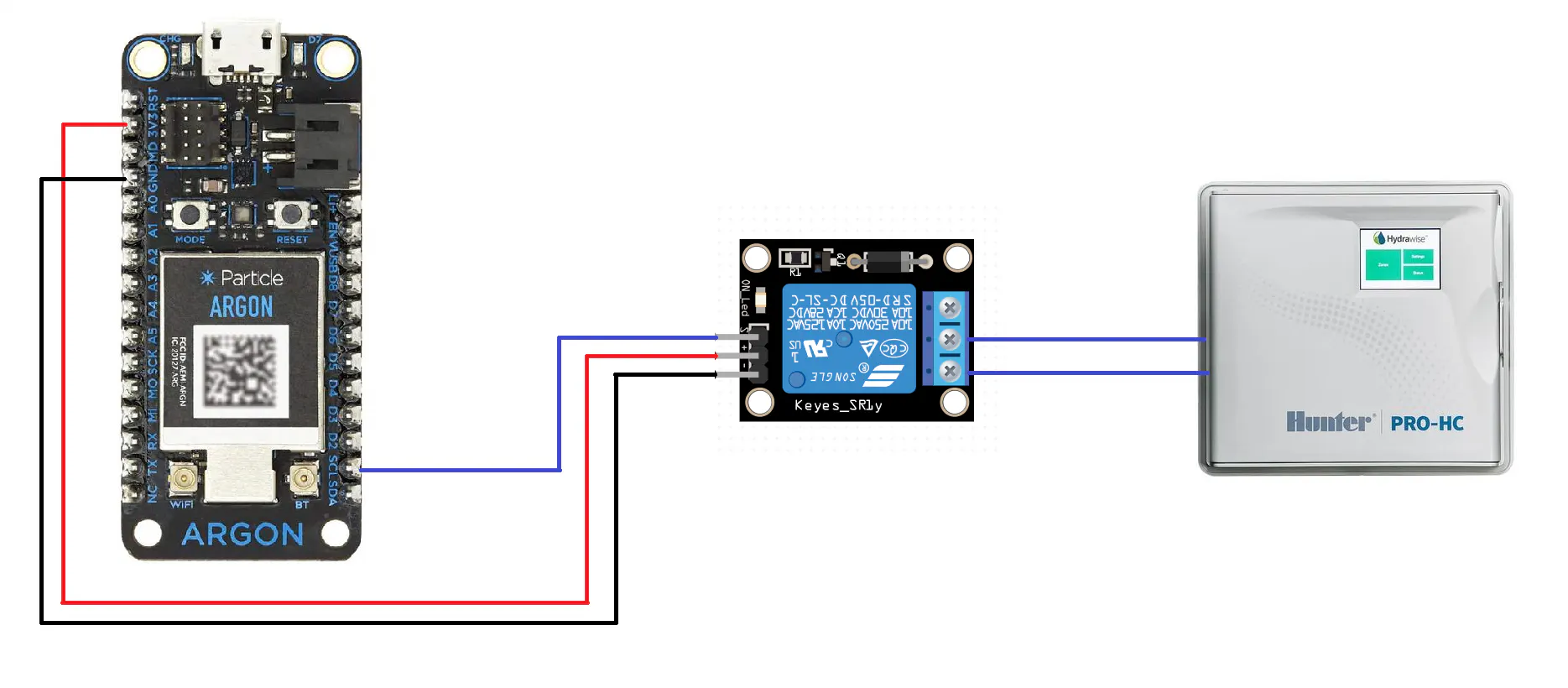### LCD Argon Schematic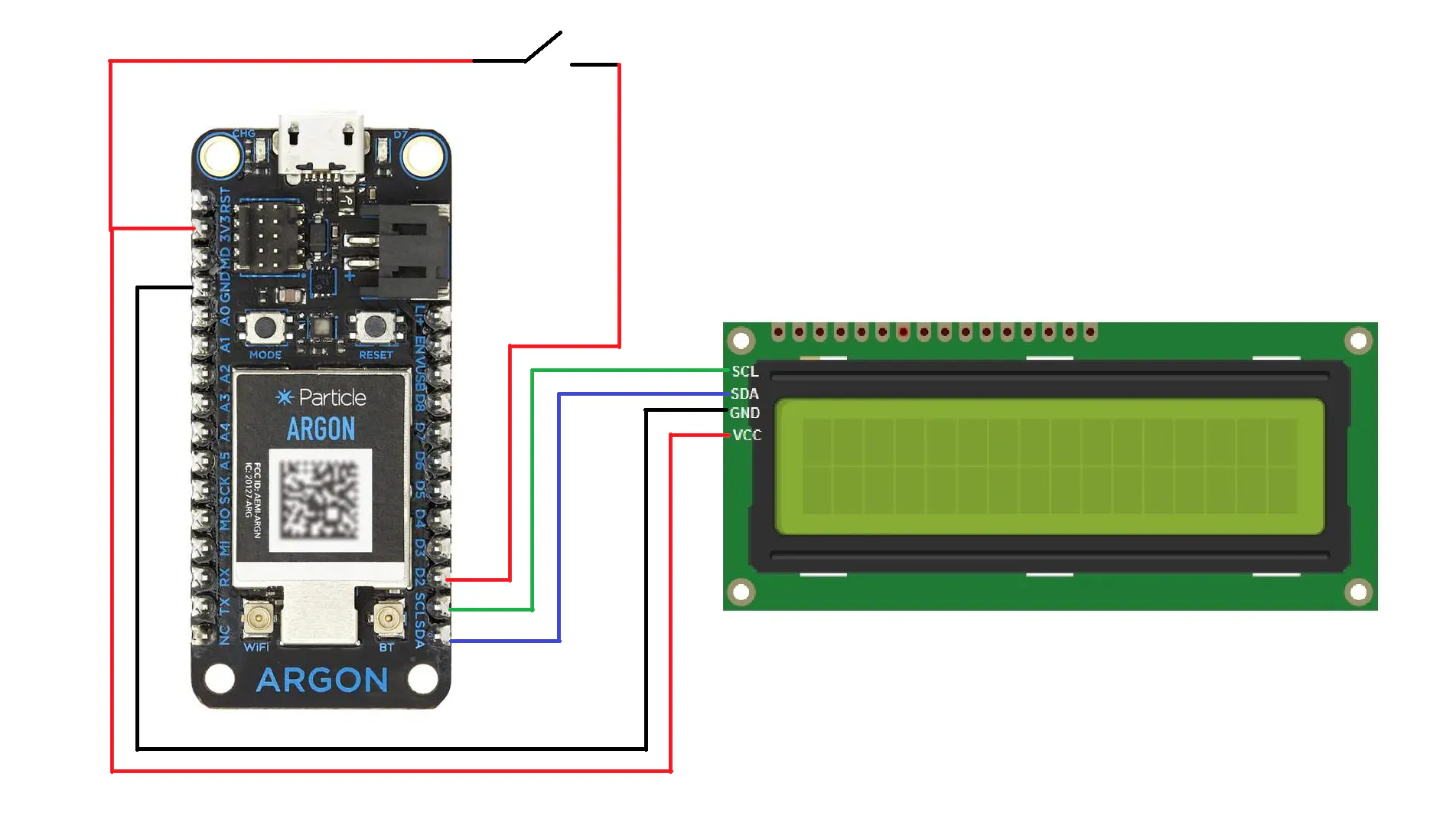## Code

### Sensor Argon Code

C/C++
```#include <JsonParserGeneratorRK.h>
#include <math.h>
#include <OneWire.h>
#include <DS18.h>
#include <iomanip>
#include <string>

// Variables for temperature reading
DS18 sensor(D2);
char temp;
float current_temp = 0;
float previous_temp = 0;
int tempcounter = 0;

//Variables for boat lift position
int BL = D4;
int lift_state = 2;//Set to arbitrary numbers to begin if statement in void boatlift
int prev_lift_state = 3;//Same as lift_state

// Variables for lake level reading
int CLK = D0;
int DT = D1;
int PrevCLKState;
int CLKState;
int counter = 0;
float Lake_level;
float Wheel_Dia = 0.5;
float lake_level_offset=97.4;
int irrigation_cutoff = 96;
int k;
float rounded_lake;
String string_lake = "";
String rounded_string_lake = "";

void setup()
{
pinMode(BL, INPUT);
pinMode(CLK, INPUT);
pinMode(DT, INPUT);

//This If else ststement checks the initial lake level offset and publishes if the lake lavel is OK for irrigation or below the irrigation cutoff
Lake_level = lake_level_offset;  //Converts rotary encoder position into lake level (ft)
string_lake = String(Lake_level);

if(lake_level_offset >= irrigation_cutoff)
{
Particle.publish("Lake Level OK", PRIVATE);
k = 0;
}
else
{
Particle.publish("Lake Level Too Low", PRIVATE);
k = 1;
}

//Initializing some particle variables (not really used)
Particle.variable("Temperature", temp);
Particle.variable("LakeLevel", string_lake);
Particle.subscribe("Is lake level OK?", lake_ok, "e00fce68d8d3966b6d563d65");
}

void loop()
{
if(tempcounter != 3000000) //300000 Fixes problem with encoder loop not being fast enought to determine directionn of rotation
{
encoder(); //Calls encoder sub program to run
}
else  //Once 300000 is reahced the temperature will be recorded and irrigstion switch code will run as well
{
boatlift();
tempSensor(); //Calls Temperature sensor program to run
irrigation(); //Calls Irrigation decision program to run
Particle.publish("Lake Level", string_lake, PRIVATE); //Publishes new lake level as an event
tempcounter = 0; //resets cycle counter to 0
}
tempcounter ++;
}

//Subprogram to read and publish an event if the boat lift has changed positions
void boatlift()
{
if(lift_state == LOW && lift_state != prev_lift_state)
{
Particle.publish("Boat Lift Raised", PRIVATE);
prev_lift_state = lift_state;
}
else if(lift_state == HIGH && lift_state != prev_lift_state)
{
Particle.publish("Boat Lift Lowered", PRIVATE);
prev_lift_state = lift_state;
}
}

//Subprogram for lake level (encoder)
void encoder()
{
CLKState = digitalRead(CLK); // Reads current state of CLK pin

if(CLKState != PrevCLKState)//Looks for a change of state
{
// CW rotation
if(digitalRead(DT) != CLKState)//If DT pin lags CLK pin (DT!=CLK) it then must be a CW rotation
{
counter --;
}
//CCW Rotation
else //If DT pin leads CLK pin (DT=CLK) it then must be a CCW rotation
{
counter ++;
}

PrevCLKState = CLKState;
Lake_level = (counter * 3.14159 * Wheel_Dia / (20 * 12)) + lake_level_offset;  //Converts rotary encoder position into lake level (ft)
//string_lake = String(round(Lake_level*10)/10.0); //Converts the lake level floating point value into a string so that it can be used in a particle variable

// char buffer;
string_lake = String(Lake_level);
rounded_lake = trunc(Lake_level*100)*.01;
rounded_string_lake = String(rounded_lake);
//rounded_string_lake = sprintf("%1.2f", Lake_level);
//Particle.publish("Rounded Lake Level", rounded_string_lake, PRIVATE); //Publishes new lake level as an event
//Particle.publish("Lake Level", string_lake, PRIVATE);
}
}

void irrigation(){

//Sends a particle publish if the water is too low or OK

if(Lake_level < irrigation_cutoff && k == 0){
Particle.publish("Lake Level Too Low",  PRIVATE);
k = 1;
}
if(Lake_level >= irrigation_cutoff && k == 1){
Particle.publish("Lake Level OK", PRIVATE);
k = 0;
}
}

//Subprogram for temperature reading
void tempSensor()
{

{
current_temp = sensor.fahrenheit();

if (abs(current_temp - previous_temp) >=0.05)
{
//snprintf(temp, sizeof(temp), "Temperature %0.2f", current_temp);
Particle.publish("Temperature", String(sensor.fahrenheit()),PRIVATE);
previous_temp = current_temp;
}
}
}

void lake_ok(const char *event, const char *data)//IDk if it is the const char event line
{
//Sends a particle publish if the water is too low or OK
if(Lake_level < irrigation_cutoff && k == 1)
{
Particle.publish("Lake Level Too Low", PRIVATE);
}
else if(Lake_level >= irrigation_cutoff && k == 0)
{
Particle.publish("Lake Level OK", PRIVATE);
}
}

void upload_data(float Lake_level, float current_temp, int lift_state)
{
JsonWriterStatic<256> jw;
{
JsonWriterAutoObject obj(&jw);

jw.insertKeyValue("Lift", lift_state);
jw.insertKeyValue("Temp", current_temp);
jw.insertKeyValue("Lake", Lake_level);

}

Particle.publish("Sensor Values", jw.getBuffer(), PRIVATE);
}
```

### LCD Argon Code

C/C++
```#include <LiquidCrystal_I2C_Spark.h>

LiquidCrystal_I2C *lcd;

int button = D2;
int button_state;
int  k = 0;

int i = 0;
const char *llevel;
const char *temp;

void setup()
{

pinMode(D2, INPUT);

Particle.subscribe("Lake Level", level , "e00fce681dc977cc0d02e49d");
Particle.subscribe("Temperature", temperature , "e00fce681dc977cc0d02e49d");

lcd = new LiquidCrystal_I2C(0x27, 16, 2);

lcd->init();
lcd->backlight();

lcd->setCursor(0 ,0 );
lcd->print("Level");
lcd->setCursor(12 ,0 );
lcd->print("FT");

lcd->setCursor(0 ,1 );
lcd->print("Temp");
lcd->setCursor(12 ,1 );
lcd->print("DegF");
}

void loop()
{

if(button_state == LOW && k == 0)
{
Particle.publish("Manual Irrigation Cancelation", PRIVATE);
k = 1;
lcd->setCursor(15 ,0 );
lcd->print("I");
delay(400);
}

else if(button_state == LOW && k == 1)
{
Particle.publish("Manual Irrigation Resume", PRIVATE);
k = 0;
lcd->setCursor(15 ,0 );
lcd->print("A");
delay(400);
}

}

void level(const char *event, const char *data)
{

lcd->setCursor(0 ,0 );
// lcd->print("Lake");
lcd->setCursor(6 ,0);
for (int i = 0; i < 5; i++) {
lcd->print(data[i]);
}

}

void temperature(const char *event2, const char *data)
{

lcd->setCursor(6 ,1 );
for (int i = 0; i < 5; i++) {
lcd->print(data[i]);
}
}
```

### Sprinkler Relay Argon Code

C/C++
```void setup() {
Particle.subscribe("Lake Level Too Low", irrigationoff , "e00fce681dc977cc0d02e49d");
Particle.subscribe("Lake Level OK", irrigationon , "e00fce681dc977cc0d02e49d");
pinMode(D0, OUTPUT);
Particle.publish("Is lake level OK?");
}

void irrigationoff(const char *event, const char *data)
{
digitalWrite(D0,HIGH);
}

void irrigationon(const char *event, const char *data)
{
digitalWrite(D0,LOW);
}

void loop() {

}
```

## Credits

### Daniel Shirilla

1 project • 0 followers